# Electronics and Communication Engineering - Exam Questions Papers

36.

The amplitude modulated wave is given by __________ e = 25(1 + 0.7 cos 5000t - 0.3 cos 1000t) x sin 5 x 106t The amplitude of carrier and sideband frequencies in magnitudes are

 A. 25, 0.7, 0.3 B. 25, 0.7, - 0.3 C. 24, 17.5, 7.5 D. 25, 8.75, 3.75

Explanation:

Modulation index is 0.7 and 0.3 so that amplitude of signal is 0.7 x 25 = 17.5 and 0.3 x 25 = 7.5

On modulation the sideband amplitude is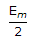.

Hence 8.75 and 3.7537.

A signal flow graph of a system is given below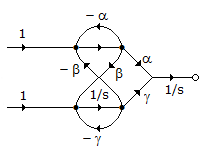The set of equations that correspond of this signal flow graph is

 A.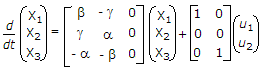B.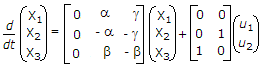C.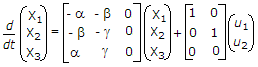D.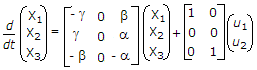Explanation: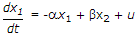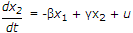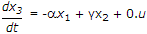.

38.

(11)2 + (11)3 = (11)?

 A. 4 B. 5 C. 6 D. not possible

Explanation:

(11)2 + (11)3 = 3 + 4 = 7 and

(11)6 = 61 + 60 = 6 + 1 = 7.

39.

If h1[n] = 3δ[n] + δ[n - 1], h2[n] = 2δ[n] + δ[n - 2]
h3[n] = δ[n] - 3δ[n - 1] + 7δ[n - 4] + 6δ[n - 6]
h[n] = h1[n] * h2[n] + h3[n] * h1[n] has value

 A. {6, 18, 7, 4, 0, - 6, 9} B. {9, 0, - 6, 21, 7, 6, 1} C. {7, - 1, 3, 1, 7, 0, 6} D. {9, - 6, 0, 1, 21, 7, 18, 6}

Explanation:

h1[n] * h2[n] + h3[n] * h1[n] = h1[n] * (h2[n] + h3[n])

Distributive property for convolution,

(h2[n] + h3[n]) = 3δ(n) - 3δ(n - 1) + δ(n - 2) + 7δ(n -4) + 6δ(n - 6) then h1(n) * (h2(n) + h3(n))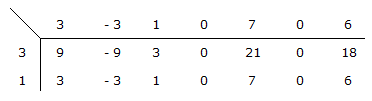{9, - 6, 0, 1, 21, 7, 18, 6}.

40.

The feedback control system shown in the given figure represents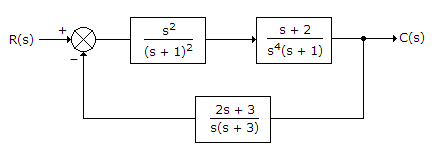A. Type 0 system B. Type 1 system C. Type 2 system D. Type 3 system

Explanation: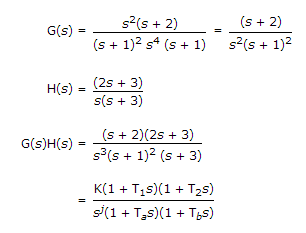j gives type of system

j = 3

given system is type 3 system.

#### Current Affairs 2022

Interview Questions and Answers